Starting with maths: Patterns and formulas

Start this free course now. Just create an account and sign in. Enrol and complete the course for a free statement of participation or digital badge if available.

Free course

# 3.5 Maths in archaeology

In several different parts of the world, footprints from prehistoric human civilisations have been found preserved in either sand or volcanic ash. From these tracks it is possible to measure the foot length and the length of the stride. These measurements can be used to estimate both the height of the person who made the footprint and also whether the person was walking or running by using the following three formulas: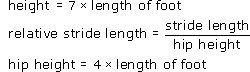Note that no units have been included in these formulas, so it is important to make sure that the same units, for example centimetres, are used throughout the calculation. If the value of the relative stride length is less than 2, the person was probably walking and if the value is greater than 2.9, the person was probably running.

## Activity 8: Footprints in the sand

From one set of footprints the length of the foot is measured as 21.8 cm and the stride length as 104.6 cm. What does the data suggest about the height and the motion of the person who made these footprints?

### Discussion

The height of the person is estimated to be 7 × 21.8 cm or approximately 153 cm.

To work out the relative stride length, we need to calculate the hip height and then substitute it into the relative stride length formula.

The hip height is 4 × 21.8 cm or 87.2 cm.

So the relative stride length =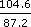= 1.2 (1 d.p).

As the relative stride length is less than 2, the person was probably walking.

Notice that the relative stride length has no units. The stride length and the hip height are both measured in cm, and when we divide one by the other, these units cancel each other out, just like cancelling numbers. If you go on to study physics or other sciences, you will find lots of examples like this.

How do you think these formulas have been derived? How reliable do you think these estimates are?

Notice that to find the relative stride length here, we tackled the problem by using the two formulas step by step, but you could also have combined these two formulas into one: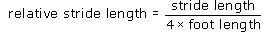and used this new formula directly.

## Activity 9: What's wrong?

A student used the formula: relative stride length =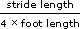to calculate the relative stride length for the person in Activity 8. Use this formula to work out the relative stride length and check that your answer agrees with the result in the last activity. The student entered the key sequence for the calculation as 104.6 ÷ 4 × 21.8. This gave an answer of approximately 570, so the student concluded that the person was probably running very fast indeed. Can you explain where the student made his mistake?

### Discussion

The calculator will perform this calculation from left to right, using the BIDMAS (Brackets, Indices, Division, Multiplication, Addition and Subtraction) rules, which treat multiplication and division as equally important. So it will first divide 104.6 by 4 to get 26.15 and then multiply by 21.8 to get approximately 570. However, this is not the correct calculation from the formula. The stride length (104.6) should be divided by (4 × foot length), so the calculation should be 104.6 ÷ (4 × 21.8) or alternatively 104.6 ÷ 4 ÷ 21.8. Enter both these expressions into your calculator directly, and check that these both do give the correct answer.

Can you explain why these two calculations are equivalent?

Note that the student should have been expecting an answer between about 0 and 5, so an answer of 570 is suspicious! Also if the student had worked out an estimate for the answer first, the mistake might have been spotted. (If you round all the values to 1 significant figure, an estimate for the answer is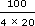which is just over 1.)

Now, have a look back over the formulas you have used so far and also any others that you might be familiar with from your home, work or hobbies. What are the common steps in using a formula? If you were asked to jot down a list of tips for using a formula for a friend, what would you say? Here are a few suggestions – feel free to add your own to the Comments section below!

## Tips for using a formula

• First, check the formula can be applied to your particular problem!

• Check what values you need to substitute and also what units these should be measured in. Convert the measurements if necessary.

• Substitute the values into the formula carefully.

• Make a rough estimate for the answer.

• Use BIDMAS to work out the resulting calculation, step by step.

• Explain your steps carefully using words like ‘substituting’ and ‘converting’.

• Check that your answer seems reasonable, both practically and from the estimate.

• Remember your concluding sentence and the units!